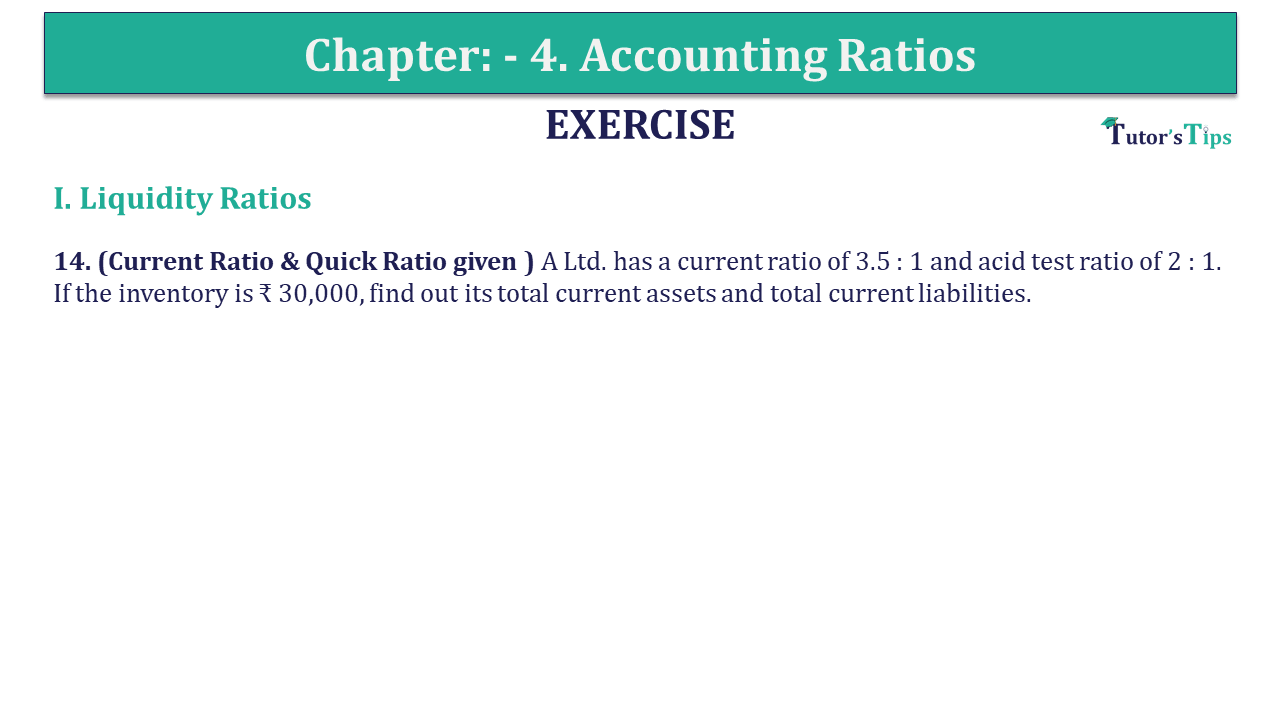# Question 15 Chapter 4 of +2-B – USHA Publication 12 ClassQ-15- CH-4 Book 2 - Usha Pub. +2 Book 2020 - Solution

Question 15 Chapter 4 of +2-B

I. Liquidity Ratios

15. (Current Ratio & Quick Ratio given ) A Ltd. has a current ratio of 3.5 : 1 and acid test ratio of 2 : 1. If the inventory is ₹ 30,000, find out its total current assets and total current liabilities.

## The solution of Question 15 Chapter 4 of +2-B: –

Solution: – (a)

 Current Ratio = 3.5 : 1 Acid Test Ratio = 2 : 1 Inventories = Current Assets –Quick Assets = 3.5 – 2 ₹ 30,000 = 1.5

 Current Assets = 30,000 X 3.5 1.5 = ₹ 70,000
 Current Liabilities = 30,000 X 3.5 1.5 = ₹ 20,000

What are Liquidity Ratios – Formulas and Examples

Comment if you have any question.

Also, Check out the solved question of previous Chapters: –